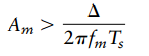Create an Account

Home / Questions / Consider a sinusoidal wave of frequency fm and amplitude Am applied to a delta modulator o...

Consider a sinusoidal wave of frequency fm and amplitude Am applied to a delta modulator of step size Show that slope overload distortion will occur if where is the sampling period What is the

Consider a sinusoidal wave of frequency fm and amplitude Am, applied to a delta modulator of step size

∆. Show that slope-overload distortion will occur ifwhere is the sampling period. What is the maximum power that may be transmitted without slope-overload distortion?

Jul 31 2020 View more View LessSubscribe To Get Solution